Exceptional Lie Algebras Next: G2 Up: The Octonions Previous: OP2 and the Exceptional Jordan Algebra

# 4. Exceptional Lie Algebras

On October 18th, 1887, Wilhelm Killing wrote a letter to Friedrich Engel saying that he had classified the simple Lie algebras. In the next three years, this revolutionary work was published in a series of papers . Besides what we now call the 'classical' simple Lie algebras, he claimed to have found 6 'exceptional' ones — new mathematical objects whose existence had never before been suspected. In fact he only gave a rigorous construction of the smallest of these. In his 1894 thesis, Cartan  constructed all of them and noticed that the two 52-dimensional exceptional Lie algebras discovered by Killing were isomorphic, so that there are really only 5.

The Killing-Cartan classification of simple Lie algebras introduced much of the technology that is now covered in any introductory course on the subject, such as roots and weights. In what follows we shall avoid this technology, since we wish instead to see the exceptional Lie algebras as octonionic cousins of the classical ones — slightly eccentric cousins, but still having a close connection to geometry, in particular the Riemannian geometry of projective planes. It is also for this reason that we shall focus on the compact real forms of the simple Lie algebras.

The classical simple Lie algebras can be organized in three infinite families: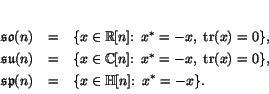The corresponding Lie groups are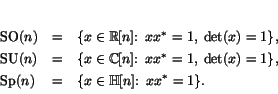These arise naturally as symmetry groups of projective spaces over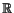,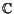, and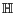, respectively. More precisely, they arise as groups of isometries: transformations that preserve a specified Riemannian metric. Let us sketch how this works, as a warmup for the exceptional groups.

First consider the projective space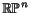. We can think of this as the unit sphere in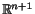with antipodal points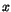and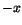identified. It thus inherits a Riemannian metric from the sphere, and the obvious action of the rotation group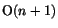as isometries of the sphere yields an action of this group as isometries ofwith this metric. In fact, with this metric, the group of all isometries ofis just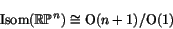where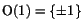is the subgroup ofthat acts trivially on. The Lie algebra of this isometry group is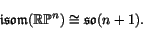The case of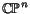is very similar. We can think of this as the unit sphere in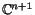with pointsand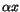identified whenever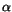is a unit complex number. It thus inherits a Riemannian metric from this sphere, and the unitary group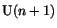acts as isometries. If we consider only the connected component of the isometry group and ignore the orientation-reversing isometries coming from complex conjugation, we have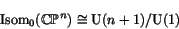where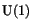is the subgroup that acts trivially on. The Lie algebra of this isometry group is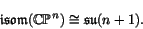The case of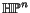is subtler, since we must take the noncommutativity of the quaternions into account. We can think ofas the unit sphere in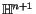with pointsandidentified wheneveris a unit quaternion, and as before,inherits a Riemannian metric. The group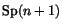acts as isometries of, but this action comes from right multiplication, so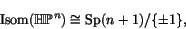since not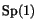but only its center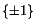acts trivially onby right multiplication. At the Lie algebra level, this gives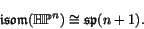For lovers of the octonions, it is tempting to try a similar construction starting with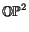. While nonassociativity makes things a bit tricky, we show in Section 4.2 that it can in fact be done. It turns out that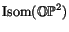is one of the exceptional Lie groups, namely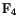. Similarly, the exceptional Lie groups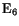,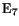andare in a certain subtle sense the isometry groups of projective planes over the algebras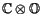,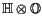and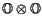. Together with, these groups can all be defined by the so-called 'magic square' construction, which makes use of much of the algebra we have described so far. We explain three versions of this construction in Section 4.3. We then treat the groups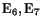andindividually in the following sections. But first, we must introduce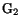: the smallest of the exceptional Lie groups, and none other than the automorphism group of the octonions.

Subsections
Next: G2 Up: The Octonions Previous: OP2 and the Exceptional Jordan Algebra